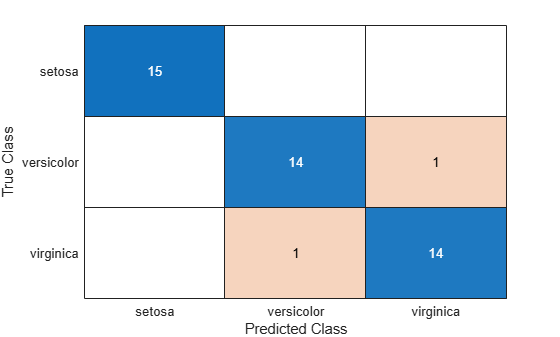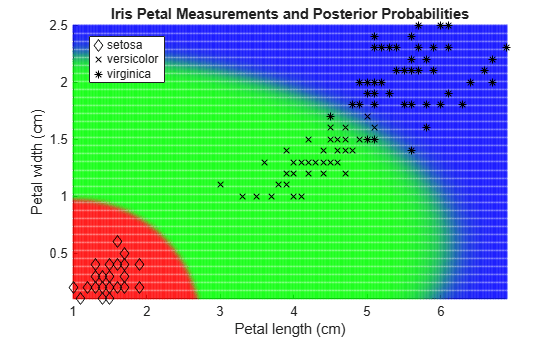# predict

Classify observations using naive Bayes classifier

## Syntax

``label = predict(Mdl,X)``
``````[label,Posterior,Cost] = predict(Mdl,X)``````

## Description

example

````label = predict(Mdl,X)` returns a vector of predicted class labels for the predictor data in the table or matrix `X`, based on the trained naive Bayes classification model `Mdl`. The trained naive Bayes model can either be full or compact.```

example

``````[label,Posterior,Cost] = predict(Mdl,X)``` also returns the Posterior Probability (`Posterior`) and predicted (expected) Misclassification Cost (`Cost`) corresponding to the observations (rows) in `Mdl.X`. For each observation in `X`, the predicted class label corresponds to the minimum expected classification cost among all classes.```

## Examples

collapse all

Load the `fisheriris` data set. Create `X` as a numeric matrix that contains four petal measurements for 150 irises. Create `Y` as a cell array of character vectors that contains the corresponding iris species.

```load fisheriris X = meas; Y = species; rng('default') % for reproducibility```

Randomly partition observations into a training set and a test set with stratification, using the class information in `Y`. Specify a 30% holdout sample for testing.

`cv = cvpartition(Y,'HoldOut',0.30);`

Extract the training and test indices.

```trainInds = training(cv); testInds = test(cv);```

Specify the training and test data sets.

```XTrain = X(trainInds,:); YTrain = Y(trainInds); XTest = X(testInds,:); YTest = Y(testInds);```

Train a naive Bayes classifier using the predictors `XTrain` and class labels `YTrain`. A recommended practice is to specify the class names. `fitcnb` assumes that each predictor is conditionally and normally distributed.

`Mdl = fitcnb(XTrain,YTrain,'ClassNames',{'setosa','versicolor','virginica'})`
```Mdl = ClassificationNaiveBayes ResponseName: 'Y' CategoricalPredictors: [] ClassNames: {'setosa' 'versicolor' 'virginica'} ScoreTransform: 'none' NumObservations: 105 DistributionNames: {'normal' 'normal' 'normal' 'normal'} DistributionParameters: {3x4 cell} ```

`Mdl` is a trained `ClassificationNaiveBayes` classifier.

Predict the test sample labels.

```idx = randsample(sum(testInds),10); label = predict(Mdl,XTest);```

Display the results for a random set of 10 observations in the test sample.

```table(YTest(idx),label(idx),'VariableNames',... {'TrueLabel','PredictedLabel'})```
```ans=10×2 table TrueLabel PredictedLabel ______________ ______________ {'virginica' } {'virginica' } {'versicolor'} {'versicolor'} {'versicolor'} {'versicolor'} {'virginica' } {'virginica' } {'setosa' } {'setosa' } {'virginica' } {'virginica' } {'setosa' } {'setosa' } {'versicolor'} {'versicolor'} {'versicolor'} {'virginica' } {'versicolor'} {'versicolor'} ```

Create a confusion chart from the true labels `YTest` and the predicted labels `label`.

`cm = confusionchart(YTest,label);`Estimate posterior probabilities and misclassification costs for new observations using a naive Bayes classifier. Classify new observations using a memory-efficient pretrained classifier.

Load the `fisheriris` data set. Create `X` as a numeric matrix that contains four petal measurements for 150 irises. Create `Y` as a cell array of character vectors that contains the corresponding iris species.

```load fisheriris X = meas; Y = species; rng('default') % for reproducibility```

Partition the data set into two sets: one contains the training set, and the other contains new, unobserved data. Reserve 10 observations for the new data set.

```n = size(X,1); newInds = randsample(n,10); inds = ~ismember(1:n,newInds); XNew = X(newInds,:); YNew = Y(newInds);```

Train a naive Bayes classifier using the predictors `X` and class labels `Y`. A recommended practice is to specify the class names. `fitcnb` assumes that each predictor is conditionally and normally distributed.

```Mdl = fitcnb(X(inds,:),Y(inds),... 'ClassNames',{'setosa','versicolor','virginica'});```

`Mdl` is a trained `ClassificationNaiveBayes` classifier.

Conserve memory by reducing the size of the trained naive Bayes classifier.

```CMdl = compact(Mdl); whos('Mdl','CMdl')```
``` Name Size Bytes Class Attributes CMdl 1x1 5575 classreg.learning.classif.CompactClassificationNaiveBayes Mdl 1x1 12900 ClassificationNaiveBayes ```

`CMdl` is a `CompactClassificationNaiveBayes` classifier. It uses less memory than `Mdl` because `Mdl` stores the data.

Display the class names of `CMdl` using dot notation.

`CMdl.ClassNames`
```ans = 3x1 cell {'setosa' } {'versicolor'} {'virginica' } ```

Predict the labels. Estimate the posterior probabilities and expected class misclassification costs.

`[labels,PostProbs,MisClassCost] = predict(CMdl,XNew);`

Compare the true labels with the predicted labels.

```table(YNew,labels,PostProbs,MisClassCost,'VariableNames',... {'TrueLabels','PredictedLabels',... 'PosteriorProbabilities','MisclassificationCosts'})```
```ans=10×4 table TrueLabels PredictedLabels PosteriorProbabilities MisclassificationCosts ______________ _______________ _________________________________________ ______________________________________ {'virginica' } {'virginica' } 4.0832e-268 4.6422e-09 1 1 1 4.6422e-09 {'setosa' } {'setosa' } 1 3.0706e-18 4.6719e-25 3.0706e-18 1 1 {'virginica' } {'virginica' } 1.0007e-246 5.8758e-10 1 1 1 5.8758e-10 {'versicolor'} {'versicolor'} 1.2022e-61 0.99995 4.9859e-05 1 4.9859e-05 0.99995 {'virginica' } {'virginica' } 2.687e-226 1.7905e-08 1 1 1 1.7905e-08 {'versicolor'} {'versicolor'} 3.3431e-76 0.99971 0.00028983 1 0.00028983 0.99971 {'virginica' } {'virginica' } 4.05e-166 0.0028527 0.99715 1 0.99715 0.0028527 {'setosa' } {'setosa' } 1 1.1272e-14 2.0308e-23 1.1272e-14 1 1 {'virginica' } {'virginica' } 1.3292e-228 8.3604e-10 1 1 1 8.3604e-10 {'setosa' } {'setosa' } 1 4.5023e-17 2.1724e-24 4.5023e-17 1 1 ```

`PostProbs` and `MisClassCost` are `10`-by-`3` numeric matrices, where each row corresponds to a new observation and each column corresponds to a class. The order of the columns corresponds to the order of `CMdl.ClassNames`.

Load the `fisheriris` data set. Create `X` as a numeric matrix that contains petal length and width measurements for 150 irises. Create `Y` as a cell array of character vectors that contains the corresponding iris species.

```load fisheriris X = meas(:,3:4); Y = species;```

Train a naive Bayes classifier using the predictors `X` and class labels `Y`. A recommended practice is to specify the class names. `fitcnb` assumes that each predictor is conditionally and normally distributed.

`Mdl = fitcnb(X,Y,'ClassNames',{'setosa','versicolor','virginica'});`

`Mdl` is a trained `ClassificationNaiveBayes` classifier.

Define a grid of values in the observed predictor space.

```xMax = max(X); xMin = min(X); h = 0.01; [x1Grid,x2Grid] = meshgrid(xMin(1):h:xMax(1),xMin(2):h:xMax(2));```

Predict the posterior probabilities for each instance in the grid.

`[~,PosteriorRegion] = predict(Mdl,[x1Grid(:),x2Grid(:)]);`

Plot the posterior probability regions and the training data.

```h = scatter(x1Grid(:),x2Grid(:),1,PosteriorRegion); h.MarkerEdgeAlpha = 0.3;```

Plot the data.

```hold on gh = gscatter(X(:,1),X(:,2),Y,'k','dx*'); title 'Iris Petal Measurements and Posterior Probabilities'; xlabel 'Petal length (cm)'; ylabel 'Petal width (cm)'; axis tight legend(gh,'Location','Best') hold off```## Input Arguments

collapse all

Naive Bayes classification model, specified as a `ClassificationNaiveBayes` model object or `CompactClassificationNaiveBayes` model object returned by `fitcnb` or `compact`, respectively.

Predictor data to be classified, specified as a numeric matrix or table.

Each row of `X` corresponds to one observation, and each column corresponds to one variable.

• For a numeric matrix:

• The variables that make up the columns of `X` must have the same order as the predictor variables that trained `Mdl`.

• If you train `Mdl` using a table (for example, `Tbl`), then `X` can be a numeric matrix if `Tbl` contains only numeric predictor variables. To treat numeric predictors in `Tbl` as categorical during training, identify categorical predictors using the `'CategoricalPredictors'` name-value pair argument of `fitcnb`. If `Tbl` contains heterogeneous predictor variables (for example, numeric and categorical data types) and `X` is a numeric matrix, then `predict` throws an error.

• For a table:

• `predict` does not support multicolumn variables or cell arrays other than cell arrays of character vectors.

• If you train `Mdl` using a table (for example, `Tbl`), then all predictor variables in `X` must have the same variable names and data types as the variables that trained `Mdl` (stored in `Mdl.PredictorNames`). However, the column order of `X` does not need to correspond to the column order of `Tbl`. `Tbl` and `X` can contain additional variables (response variables, observation weights, and so on), but `predict` ignores them.

• If you train `Mdl` using a numeric matrix, then the predictor names in `Mdl.PredictorNames` must be the same as the corresponding predictor variable names in `X`. To specify predictor names during training, use the '`PredictorNames`' name-value pair argument of `fitcnb`. All predictor variables in `X` must be numeric vectors. `X` can contain additional variables (response variables, observation weights, and so on), but `predict` ignores them.

Data Types: `table` | `double` | `single`

Notes:

• If `Mdl.DistributionNames` is `'mn'`, then the software returns `NaN`s corresponding to rows of `X` that contain at least one `NaN`.

• If `Mdl.DistributionNames` is not `'mn'`, then the software ignores `NaN` values when estimating misclassification costs and posterior probabilities. Specifically, the software computes the conditional density of the predictors given the class by leaving out the factors corresponding to missing predictor values.

• For predictor distribution specified as `'mvmn'`, if `X` contains levels that are not represented in the training data (that is, not in `Mdl.CategoricalLevels` for that predictor), then the conditional density of the predictors given the class is 0. For those observations, the software returns the corresponding value of `Posterior` as a `NaN`. The software determines the class label for such observations using the class prior probability stored in `Mdl.Prior`.

## Output Arguments

collapse all

Predicted class labels, returned as a categorical vector, character array, logical or numeric vector, or cell array of character vectors.

The predicted class labels have the following:

• Same data type as the observed class labels (`Mdl.Y`). (The software treats string arrays as cell arrays of character vectors.)

• Length equal to the number of rows of `Mdl.X`.

• Class yielding the lowest expected misclassification cost (`Cost`).

Class Posterior Probability, returned as a numeric matrix. `Posterior` has rows equal to the number of rows of `Mdl.X` and columns equal to the number of distinct classes in the training data (`size(Mdl.ClassNames,1)`).

`Posterior(j,k)` is the predicted posterior probability of class `k` (in class `Mdl.ClassNames(k)`) given the observation in row `j` of `Mdl.X`.

Expected Misclassification Cost, returned as a numeric matrix. `Cost` has rows equal to the number of rows of `Mdl.X` and columns equal to the number of distinct classes in the training data (`size(Mdl.ClassNames,1)`).

`Cost(j,k)` is the expected misclassification cost of the observation in row `j` of `Mdl.X` predicted into class `k` (in class `Mdl.ClassNames(k)`).

collapse all

### Misclassification Cost

A misclassification cost is the relative severity of a classifier labeling an observation into the wrong class.

Two types of misclassification cost exist: true and expected. Let K be the number of classes.

• True misclassification cost — A K-by-K matrix, where element (i,j) indicates the cost of classifying an observation into class j if its true class is i. The software stores the misclassification cost in the property `Mdl.Cost`, and uses it in computations. By default, `Mdl.Cost(i,j)` = 1 if `i``j`, and `Mdl.Cost(i,j)` = 0 if `i` = `j`. In other words, the cost is `0` for correct classification and `1` for any incorrect classification.

• Expected misclassification cost — A K-dimensional vector, where element k is the weighted average cost of classifying an observation into class k, weighted by the class posterior probabilities.

`${c}_{k}=\sum _{j=1}^{K}\stackrel{^}{P}\left(Y=j|{x}_{1},...,{x}_{P}\right)Cos{t}_{jk}.$`

In other words, the software classifies observations into the class with the lowest expected misclassification cost.

### Posterior Probability

The posterior probability is the probability that an observation belongs in a particular class, given the data.

For naive Bayes, the posterior probability that a classification is k for a given observation (x1,...,xP) is

`$\stackrel{^}{P}\left(Y=k|{x}_{1},..,{x}_{P}\right)=\frac{P\left({X}_{1},...,{X}_{P}|y=k\right)\pi \left(Y=k\right)}{P\left({X}_{1},...,{X}_{P}\right)},$`

where:

• $P\left({X}_{1},...,{X}_{P}|y=k\right)$ is the conditional joint density of the predictors given they are in class k. `Mdl.DistributionNames` stores the distribution names of the predictors.

• π(Y = k) is the class prior probability distribution. `Mdl.Prior` stores the prior distribution.

• $P\left({X}_{1},..,{X}_{P}\right)$ is the joint density of the predictors. The classes are discrete, so $P\left({X}_{1},...,{X}_{P}\right)=\sum _{k=1}^{K}P\left({X}_{1},...,{X}_{P}|y=k\right)\pi \left(Y=k\right).$

### Prior Probability

The prior probability of a class is the assumed relative frequency with which observations from that class occur in a population.

## Alternative Functionality

To integrate the prediction of a naive Bayes classification model into Simulink®, you can use the ClassificationNaiveBayes Predict block in the Statistics and Machine Learning Toolbox™ library or a MATLAB® Function block with the `predict` function. For examples, see Predict Class Labels Using ClassificationNaiveBayes Predict Block and Predict Class Labels Using MATLAB Function Block.

When deciding which approach to use, consider the following:

• If you use the Statistics and Machine Learning Toolbox library block, you can use the Fixed-Point Tool (Fixed-Point Designer) to convert a floating-point model to fixed point.

• Support for variable-size arrays must be enabled for a MATLAB Function block with the `predict` function.

• If you use a MATLAB Function block, you can use MATLAB functions for preprocessing or post-processing before or after predictions in the same MATLAB Function block.

## Version History

Introduced in R2014b